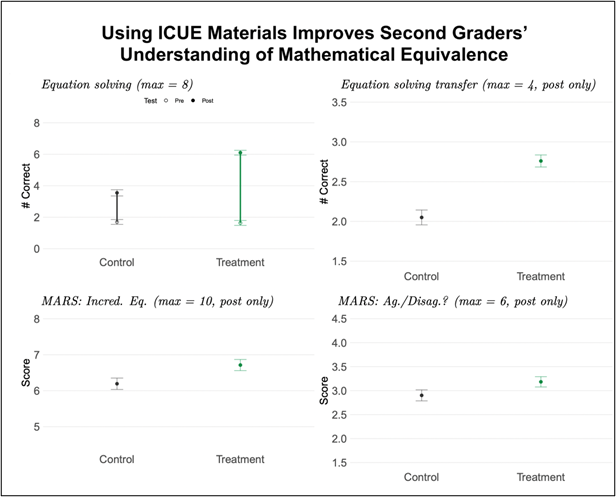Jodi L. Davenport, Yvonne Kao, Kristen Johannes, Caroline Byrd Hornburg, and Nicole M. McNeil

Does the ICUE intervention improve math learning?

Yes, second grade students in classrooms using the Improving Children’s Understanding of Equivalence (ICUE) materials and lessons scored higher on measures related to mathematical equivalence, including equation solving and conceptual problem solving. These higher scores came with no observable trade-offs in computational fluency.

The current study shows that modifications to arithmetic practice in early elementary school can help children construct a formal understanding of mathematical equivalence, a key concept for later algebra learning.The upper left chart shows that treatment students made larger gains on equation solving from pretest to posttest. The remaining charts show higher posttest scores on measures of mathematical equivalence.

Why is mathematical equivalence important?

Understanding mathematical equivalence, the relationship between two interchangeable quantities, is fundamental to algebraic reasoning. However, most elementary students struggle to grasp equivalence because traditional instruction perpetuates unhelpful conceptions.

What are the features of the ICUE intervention?

The Improving Children’s Understanding of Equivalence (ICUE) intervention integrates four research-based strategies to improve outcomes for second grade students: (1) introducing the equal sign before arithmetic, (2) non-traditional arithmetic practice, (3) concreteness fading exercises, and (4) comparison and explanation.

How did we do this study?

We conducted a large-scale randomized control trial that meets Tier 1 ESSA evidence standards. 132 second grade teachers and 3006 students from 53 California public schools were randomly assigned to the ICUE treatment condition (i.e., ICUE lessons and materials) or the active control condition (i.e., materials that focus on non-traditional arithmetic practice alone). Teachers used their assigned materials as a supplement to their regular mathematics lessons for 15-20 minutes, twice a week, over approximately 16 weeks. To measure the effect of the ICUE intervention, we compared post-tests scores of students in classrooms using ICUE materials and lessons (treatment) with those using non-traditional arithmetic materials (control). Our findings reflect an analytic sample of 121 teachers that completed all study activities.

For more information about the ICUE intervention, including freely available materials and videos describing ICUE’s four research-based strategies, visit here.